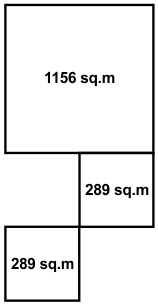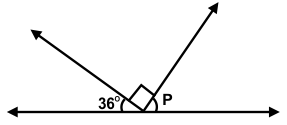﻿ Maths Olympiad (CMO) Previous Year Paper for Class 5

# CREST Mathematics Olympiad Class 5 Previous Year Papers

CREST Mathematics Olympiad Previous Year Paper PDF for Class 5:

## Syllabus:

Section 1: Numerals, Number Names and Number Sense (7 and 8 digit numbers), Computation Operations, Fractions and Decimals, Measurement of Length, Weight, Capacity, Volume, Time, Temperature and Money, Conversions, Geometrical Shapes and Solids, Angles, Perimeter of Various Shapes & Area of Rectangle and Square, Symmetry, Data Handling.

Achievers Section: Higher Order Thinking Questions - Syllabus as per Section 1

 Q.1 How many times during a day the hands of a clock make a straight line?
 Q.2 Find the perimeter of the given figure. The figure is made up of squares with the areas as mentioned:Q.3 The mixed fraction 5 4/7 can be expressed as:
 Q.4 Find the measure of ∠p in the given figure:Q.5 A car covers 24.5 km in 4.2 L of petrol. How much distance will it cover using 2 L of petrol?
 Q.6 For a project, Reh has to go and live in a different city. He left on 14th March and stayed there for 59 days (including the day he left). When did he come back?
 Q.7 The LCM of a and b is 220. Which of the following can be the HCF of a and b?
 Q.8 While cooking a curry, the mass of it reduced from 1 kg to 850 g. Find the % reduction in the weight of the curry.
 Q.9 Where will the hour hand of a clock stop if it shows 8 o'clock and turns one right angle clockwise?
 Q.10 James bought two watches for his parents. The cost of his mother's watch is 7990 cents more than his father's watch. If he spent a total of 28,000 cents on the watches, then find the cost of his mother's watch.

 Q.1 d Q.2 d Q.3 b Q.4 b Q.5 b Q.6 b Q.7 c Q.8 c Q.9 b Q.10 d

Students can download the CREST Mathematics Olympiad previous year paper pdf for class 5 from this page. Each question in the paper carries 1 mark. The answer key for all the questions is also provided on the last page.

To prepare for the CREST Mathematics Olympiad exam, students must refer to the previous years’ papers. The CREST Mathematics Olympiad previous year paper for class 5 will give students a general idea of the question types, exam format and marks distribution.